Courses

# Depression in Freezing Point JEE Notes | EduRev

## JEE : Depression in Freezing Point JEE Notes | EduRev

The document Depression in Freezing Point JEE Notes | EduRev is a part of JEE category.
All you need of JEE at this link: JEE

THIRD COLLIGATIVE PROPERTY
Depression in Freezing Point
Freezing point of a substance is the temperature at which it's liquid and the solid phases have the same vapour pressure.

Key concept: As we already know from the definition of colligative properties that when a solution freezes, it is only the pure solvent which separated out in the solid form. It is this that causes a depression in the freezing point.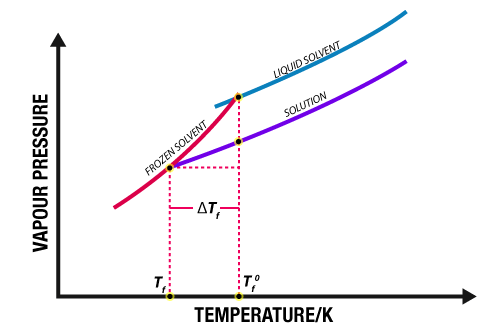Fig: Depression in freezing point.

If the difference between the freezing point of the solvent and the solution is represented as ΔTf,
Elevation of boiling point, (ΔTb) = Boiling point of the solution - Boiling point of pure solvent
= Tb -Tb˚
Experimentally, it is observed that Depression in Freezing point is directly proportional to molal concentration of solute dissolved in definite amount of the solvent.
ΔTf ∝ molality
ΔTf = Kf × molality   .....(1)
ΔT= Kf × m
Where Kf is proportionality constant and is known as the molal Depression point constant or the Cryoscopic constant. It has the units of degree kg mol-1 or K kg mol-1.
Molality can be written as
Molality (m) = Number of moles of Solute/Mass of Solvent in Kg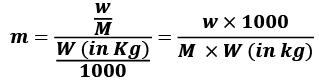w = mass of solute in grams
M = molecular wt of solute
W = mass of solvent in gram.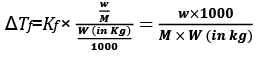Significance of Kf
ΔT= Kfm. So, if m = 1 i.e. one mole of solute is dissolved in one kg of the solvent, then, ΔTf = Kf.
Thus, the molal freezing point depression constant is equal to the depression in the freezing point produced when one mole of solute is dissolved in 1 kg of the solvent.
The values of molal elevation constant (Kb) for some common solvents are given in Table.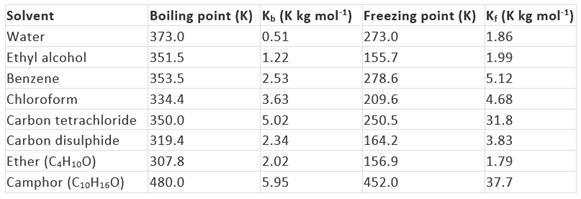Kb and Kf can be obtained from the following relationships: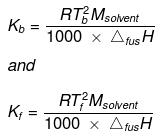Where, Tb = boiling point of the solvent,
T= freezing point of the solvent,
Msolvent = molar mass of the solvent,
ΔvapH = enthalpy of vaporization and
ΔfusH = enthalpy of fusion.

Key concept: Camphor is commonly used in determining the molecular mass of a solute because of its very high cryoscopic constant (Kf).

Example 1. An aqueous solution of a non-volatile solute boils at 100.17°C. At what temperature would it freeze? (Kb = 0.52 K kg mol - 1 and Kf = 1.88 K kg mol - 1).
Solution.
ΔTb = 0.17°C
m = ΔTb/K= 0.17/0.52
= 0.327 molal,
ΔTf = Kf × molality
ΔTf = 1.86 × 0.327 = 0.608
Freezing point of the solution is - 0.608°C.

Example 2. 1.4 g of (acetone dissolved) in 100 g of benzene gave a solution which freezes at 277.12 K. Pure benzene freezes at 278.4 . 2.8 g of a solid (A) dissolved in 100 g of benzene gave a solution which froze at 277.76 K. Calculate the molecular weight of (A).
Solution.

For acetone solute + Benzene solvent mixture:
ΔT = K'f × 1000 × w/M × W
(278.40 − 277.12) = 1000 × Kf × 1.4/100 × 58
or 1.28 = 1000 × Kf × 1.4/100 × 58 ...(i)
For solute (A) + Benzene mixture (Let M be the molecular Weight of A)
(278.40 − 277.76) = 1000 × Kf × 2.8/100 × M ...(ii)
By Eqs. (i) and (ii)
M = 232g

Example 3. Ethylene glycol (HOH2C - CH2OH) is used as an antifreeze for water to be used in car radiators in cold places. How much ethylene glycol should be added to 1 kg of water to prevent it from freezing at -10°C? Molal depression constant of water is 1.86 K kg mol-1
Solution.
Mass of water (solvent), w1 = 1 kg
ΔTf = 10°C Kf = 1.86 K kg mol-1
Mass of ethylene glycol required, w2 = ?
Molar mass of ethylene glycol = (12 + 3 +16) × 2 g/mol = 62 g/mol
We know, ΔTf = Kf(w2/62)/w1 or 10 = 1.86 × w2/62 x 1
This gives, w2 = 10 × 62 × 1/1.86 = 333.3 g

Example 4. Normal freezing point of a solvent is 150°C. A 0.5 molal solution of urea in the above solvent causes a freezing point depression of two degrees. Calculate the molal depression constant
Solution.
We know, ΔTf = Kfm
So, Kf = ΔTf/m = 2 deg/0.5 mol Kg-1 = 4 deg. kg mol-1

Example 5. A solution of urea in water freezes at 0.400°C. What will be the boiling point of the same solution if the depression and elevation constants for water are 1.86 deg kg mol-1 and 0.512 deg kg mol-1 respectively? Solution: We have the relationships:
Solution.
ΔTb = Kbn2/w1 ...(i)
And ΔTf = Kfn2/w1 ...(ii)
Where, n2 is the number of moles of the solute, and w1 is the mass of solvent in kg.
Dividing equation (i) by equation (ii),
ΔTb/ΔTf = Kb/Kf
or,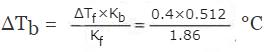= 0.11°C
Then, Boiling point of the urea solution = 100°C  + 0.11°C = 100.11°C.

TRY YOURSELF!
Q.1. What weight of glycerol would have to be added in 1000 g of water in order to lower its freezing point by 10°C? Kf for water is 1.86 K mol-1 kg.
Ans.
w = 494.6 g

Q.2. How much ethyl alcohol must be added to 1.00 litre of water so that the solution will freeze at 14°F? (Kf for water = 1.86°C/mol)
Ans. w = 247.31 g

Offer running on EduRev: Apply code STAYHOME200 to get INR 200 off on our premium plan EduRev Infinity!

,

,

,

,

,

,

,

,

,

,

,

,

,

,

,

,

,

,

,

,

,

;